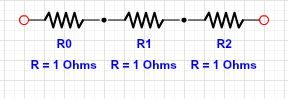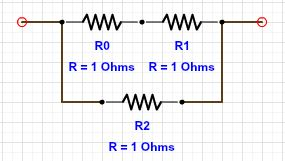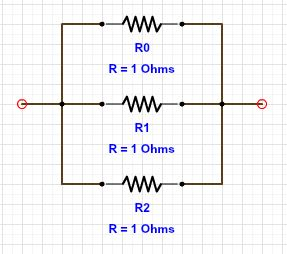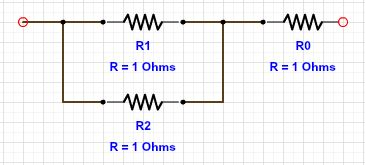# Product of equivalent resistances

Circuit: 1Circuit: 2Circuit: 3Circuit: 4In all the above circuits, there are three resistors each of resistance $$1 \Omega$$. Let $$R_{eq_1}$$, $$R_{eq_2}$$, $$R_{eq_3}$$ and $$R_{eq_4}$$ be the equivalent resistances of the circuit $$1$$, $$2$$, $$3$$ and $$4$$ respectively. Find $R_{eq_1} \times R_{eq_2} \times R_{eq_3} \times R_{eq_4}$

×

Problem Loading...

Note Loading...

Set Loading...